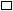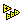# Guidelines for Using LabVIEW MathScript in Real-Time Applications (MathScript RT Module)

LabVIEW 2012 MathScript RT Module Help

Edition Date: June 2012

Part Number: 373123C-01

»View Product InfoDownload Help (Windows Only)

You can deploy VIs that contain LabVIEW MathScript Nodes with user-defined functions and certain built-in MathScript functions to RT targets. However, depending on the functions, data types, and syntax you use, LabVIEW might execute code at run time that can cause increased jitter and unbounded execution times in the real-time application. When determinism in the application is important, use the guidelines in the following sections, which are based on National Instruments testing, to reduce jitter and achieve bounded execution times for real-time applications that contain MathScript Nodes.

In addition to following these MathScript guidelines, practice techniques for reducing jitter in LabVIEW applications. Also, you can use members of the rt class of MathScript functions to perform debugging and isolate sources of jitter on RT targets.

## Setting Up MathScript NodesPlace MathScript Nodes in a time-critical VI or a timed structure. Details You must place MathScript Nodes in a time-critical VI or a Timed Loop or Timed Sequence structure to ensure the MathScript Nodes execute with the appropriate priority. The time-critical priority of VIs preempts all other priorities. Similarly, timed structures allow you to control the priority with which the MathScript Node executes relative to other timed structures on the block diagram. You also can place a subVI that contains a MathScript Node in a timed structure. If you place a MathScript Node in a timed structure, you must configure the structure to preallocate clones for instances of the MathScript Node you call within the structure. Right-click the timed structure and select Shared Clone Allocation»Preallocate from the shortcut menu to enable clone preallocation. The Timed Loop or Timed Sequence structure displays anicon to indicate that LabVIEW is preallocating clones for any reentrant VI in the structure. If you place a subVI that contains a MathScript Node in a timed structure, you must configure the subVI and all other VIs in the hierarchy to be reentrant on the Execution page of the VI Properties dialog box. For example, if you place a subVI in a timed structure and that subVI calls a subVI that contains a MathScript Node, you must configure both VIs to be reentrant.Disable nonessential options, such as debugging, for VIs that contain MathScript Nodes. Details In a VI with a MathScript Node, select File»VI Properties to display the VI Properties dialog box and select Execution from the Category pull-down menu. Remove checkmarks from the Allow debugging and Auto handle menus at launch checkboxes. You also can right-click a VI in the Project Explorer window and select Properties from the shortcut menu to launch the VI Properties dialog box. When you disable these options, VIs use less memory, compile more quickly, and exhibit less jitter.Avoid coercion dots on MathScript Node output terminals. Details LabVIEW determines the data types of variables in MathScript Nodes at edit time. If you configure the output terminal of a variable manually using a different data type than the data type LabVIEW determines, LabVIEW coerces the value to the data type you select when possible and places a coercion dot on the output terminal. Coercion dots can indicate points where the code uses more memory and increases its run time. To eliminate coercion dots, right-click an output terminal and select Choose Data Type»Auto Select Type from the shortcut menu to configure the terminal with the data type LabVIEW determines. If LabVIEW can determine the data type of a variable only at run time, the Auto Select Type option is dimmed, which indicates the data type of the variable is not suitable for deterministic applications.

## Writing MathScriptsPlace a semicolon after each line of script.

Details

When you place a semicolon after a line of script, MathScript does not display the output for that command. Not using semicolons causes unbounded execution times for the script.Note  You do not need to place semicolons after the first lines of for and while loops or switch and if-else statements, or after else and end in these constructs.Write scripts and user-defined functions you use within time-critical VIs and timed structures with recommended syntax.

Details

National Instruments has tested a subset of syntax possible in MathScript, such as matrix operations and loops, and found that this syntax typically executes in a bounded amount of time when used according to the guidelines in this topic. Other syntax might cause increased jitter and unbounded execution times in the application.Make sure inputs to operators are of the smallest possible data type. Also, make sure values are the smallest possible shape when you replace a portion of a vector or matrix with another value.

Details

For certain operations, LabVIEW might not return the smallest possible data type. For example, if you multiply a row vector and a column vector, LabVIEW might return a one-element matrix that contains the result rather than a scalar number because the result of multiplying two vectors might be a matrix. Index the scalar result from the matrix as shown in the following example:

a = ones(1, 3);
b = ones(3, 1);
c = a * b;
d = c(1) + 1.5;

To display the data type of a variable, enable data type highlighting or click outside the MathScript Node and then move the cursor over a variable in the script to display the data type in the Context Help window.Preallocate arrays that you define in a loop, and avoid resizing arrays inside loops.

Details

Do not change the size of arrays inside for and while loops. For example, avoid syntax that resizes arrays as a loop iterates, such as the following example:

a = [];
for i = 1:5
a = [a, i];
end

Instead of building an array in a loop, use the ones or zeros functions to preallocate the maximum number of elements you might need, and then replace the elements as shown in the following example:

a = zeros(1, 5);
for i = 1:5
a(i) = i;
end

If you do create an array inside a loop, do not use the array outside of the loop or during a subsequent iteration of the loop. For example, in the following script, temp is defined on the first iteration of the for loop and then used on the second and third iterations:

a = zeros(2, 5);
for i = 1:3
if i == 1
temp = [2; 2];
else
temp = 2 * temp;
a(:, i) = temp;
end
end

However, you can use syntax similar to the following example, where temp is defined and used on each iteration of the loop and the same array is not used in multiple iterations:

a = zeros(2, 5);
for i = 1:3
temp = [i; i + 1];
a(:, i) = temp;
endDefine matrices with scalar elements only. Do not use vectors or other matrices to define a matrix.

Details

For example, if you shift the order of columns in a matrix, do not redefine the matrix with the column vectors from the original matrix, as shown in the following example:

a = [1, 4, 7; 2, 5, 8; 3, 6, 9];
a = [a(:, 2:3), a(:, 1)];

Instead, you can index the columns from the original matrix to reshape the matrix, as shown in the following example:

a = [1, 4, 7; 2, 5, 8; 3, 6, 9];
temp = a(:, 1);
a(:, 1:2) = a(:, 2:3);
a(:, 3) = temp;When you specify a start:stop range to index a vector or matrix, explicitly define the range rather than defining the range in a variable.

Details

For example, do not use the following syntax to return the second through fourth elements of the vector:

a = [1, 4, 8, 3, 9, 12];
b = 2:4;
a(b);

Instead, use syntax such as a(2:4); or the following example to return the same range of three elements from the vector:

a = [1, 4, 8, 3, 9, 12];
b = 2;
a(b:b + 2);Avoid logical indexing operations.

Details

For example, do not use the following syntax, which replaces all values less than 5 in the matrix with 1:

a = [1, 4, 8; 3, 9, 12];
a(a < 5) = 1;

Instead, use the following syntax to perform the same operation without logical indexing:

a = [1, 4, 8; 3, 9, 12];
[n, m] = size(a);
for(i = 1:(n * m))
if(a(i) < 5)
a(i) = 1;
end
endDo not resize matrices during replace-indexing operations.

Details

Do not grow the size of a matrix by indexing beyond the size of the matrix. For example, avoid the following syntax, which adds a fourth column to the existing 3-by-3 matrix:

a = zeros(3, 3);
a(:, 4) = 0;

Also, do not use the [] operator to contract a matrix. Avoid syntax such as the following, which removes the second row of the existing matrix:

b = [4, 2; 7, 3];
b(2, :) = [];

## Using Built-in MathScript FunctionsWithin time-critical VIs and timed structures, call only MathScript functions suitable for deterministic applications and use values of appropriate data types as inputs to the functions. Details National Instruments has tested a subset of MathScript functions and found that the functions typically execute in a bounded amount of time when used according to the guidelines in this topic. Ensure inputs to these functions are of the data types recommended in the help topic for each function so LabVIEW does not coerce the input values.Avoid using certain functions that cause unbounded execution times on RT targets. Details Although you can deploy certain functions to RT targets, execution of those functions at run time can cause increased jitter and unbounded execution times in the real-time application. Avoid using these functions in MathScript Nodes and user-defined functions.